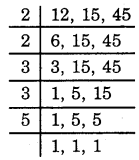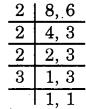# MCQ Questions for Class 6 Maths Chapter 3 Playing with Numbers with Answers

Students can access the NCERT MCQ Questions for Class 6 Maths Chapter 3 Playing with Numbers Pdf free download aids in your exam preparation and you can get a good hold of the chapter. Use MCQ Questions for Class 6 Maths with Answers during preparation and score maximum marks in the exam. Students can download the Playing with Numbers Class 6 MCQs Questions with Answers from here and test their problem-solving skills. Clear all the fundamentals and prepare thoroughly for the exam taking help from Class 6 Maths Chapter 3 Playing with Numbers Objective Questions.

## Playing with Numbers Class 6 MCQs Questions with Answers

Students are advised to solve Playing with Numbers Multiple Choice Questions of Class 6 Maths to know different concepts. Practicing the MCQ Questions on Playing with Numbers Class 6 with answers will boost your confidence thereby helping you score well in the exam.

Explore numerous MCQ Questions of Playing with Numbers Class 6 with answers provided with detailed solutions by looking below.

Question 1.
The LCM of 12 and 16 is:
(a) 32
(b) 60
(c) 48
(d) none of these

Explanation: 48LCM = 2 × 2 × 2 × 2 × 3 = 48

Prime factors of 38 are 2, 19.

Question 2.
What are the numbers which have more than two factors called?
(a) Even numbers
(b) Prime numbers
(c) Composite numbers
(d) Odd numbers

Question 3.
The sum of two odd and one even numbers is
(a) Even
(b) Prime
(c) Composite
(d) Odd

Question 4.
The number which is divisible by 5 is:
(a) 422
(b) 423
(c) 424
(d) 425

Explanation: 425, a number is divisible by 5 if its unit’s digit is either 0 or 5.

Question 5.
The HCF of 12 and 40 is:
(a) 3
(b) 4
(c) 5
(d) 6

Explanation: 4,
2 × 2 × 3
HCF = 2 × 2 = 4
40 = 2 × 2 × 2 × 5

Again, all the prime numbers you used to divide above are the Prime Factors of 144.

Question 6.
Every ______ of a number is greater than or equal to that number.
(a) factor
(b) number
(c) multiple
(d) none to these

Question 7.
What is the H.C.F. of two co-prime numbers?
(a) 1
(b) 0
(c) 2
(d) 4

Question 8.
The LCM of 12, 15, 45 is:
(a) 170
(b) 180
(c) 190
(d) 200

Explanation: 180LCM = 2 × 2 × 3 × 3 × 5 = 180

Question 9.
Find the HCF of 27, 63.
(a) 2
(b) 6
(c) 3
(d) 9

Question 10.
______ is the smallest prime number which is even.
(a) 2
(b) 4
(c) 3
(d) 1

Question 11.
If a number is divisible by 10, then which of the following can be its one’s digit?
(a) 2
(b) 3
(c) 4
(d) 0

Question 12.
The value of 10 + 40 ÷ 8 × 2 – 9 is:
(a) 9
(b) 10
(c) 11
(d) 12

Explanation: 10 + 40 ÷ 8 × 2 – 9 = 10 + 5 × 2 – 9 = 10 + 10 – 9 = 20 – 9 = 11 (BODMAS Rule)

Question 13.
The number which is divisible by 3 is:
(a) 135
(b) 136
(c) 137
(d) 139

Explanation: 135, 1 + 3 + 5 = 9 which is divisible by 3 therefore no. is divisible.

Question 14.
The only prime number which is also even is
(a) 6
(b) 1
(c) 2
(d) 4

Question 15.
Which of the following is the L.C.M. of 36 and 72?
(a) 36
(b) 72
(c) 108
(d) 2

Question 16.
A prime number is a number which:
(a) is divisible by 2
(b) is not divisible by 2
(c) has no factors
(d) has exactly two factors

Answer: (d) has exactly two factors
Explanation: Exactly two factors are 1 and the number itself.

Question 17.
Which of them is composite number?
(a) 6
(b) 5
(c) 7
(d) 3

Question 18.
Which of them is prime number?
(a) 3
(b) 6
(c) 9
(d) 8

Question 19.
The value of 18 – 3 × 4 + 1 is:
(a) 61
(b) 75
(c) 7
(d) none of these

Explanation: 7, 18 – 3 × 4 + 1 = 18 – 12 + 1 = 19 – 12 = 07 (BODMAS Rule)

Question 20.
Prime factors of 45 are:
(a) 5, 9
(b) 3, 15
(c) 3, 3, 5
(d) none of these

Explanation: 45 = 3 × 3 × 5.

Question 21.
Which of the following is the prime factorisation of 140?
(a) 2 × 2 × 7
(b) 2 × 2 × 5
(c) 2 × 2 × 5 × 7
(d) 2 × 2 × 5 × 7 × 3

Answer: (c) 2 × 2 × 5 × 7

Question 22.
Which is the number that is neither prime nor composite?
(a) 0
(b) 1
(c) 2
(d) 5

Question 23.
The common factors of 24 and 40 are:
(a) 1, 3, 4, 8
(b) 1, 2, 4, 8
(c) 1, 4, 5, 9
(d) none of these

Answer: (b) 1, 2, 4, 8
Explanation: 1, 2, 4, 8. 24 and 40 are both divisible by 1, 2, 4, 8.

Question 24.
Value of 30 + 14 ÷ 2 is:
(a) 22
(b) 29
(c) 37
(d) none of these

Explanation: 37, 30 + 14 ÷ 2 = 30 + 7 = 37 (By BODMAS Rule)

Question 25.
901153 is divisible by ___.
(a) 3
(b) 5
(c) 11
(d) 7

Question 26.
If a number is divisible by 2 and 3 both then is divisible by
(a) 3
(b) 6
(c) 5
(d) 7

Question 27.
Which of the following numbers is a prime number?
(a) 10
(b) 11
(c) 12
(d) 15

Explanation: 11 has factors 1 and 11 only.

Question 28.
The smallest prime number is:
(a) 1
(b) 2
(c) 3
(d) none of these

Explanation: Prime numbers start from the number 2.

Question 29.
Which of these numbers is a factor of every number?
(a) 0
(b) 1
(c) 2
(d) 4

Question 30.
Number of factors of a given number are _______.
(a) finite
(b) infinite
(c) 3
(d) 2

Question 31.
What is the H.C.F. of 120, 144 and 216?
(a) 38
(b) 24
(c) 120
(d) 144

Question 32.
Every prime number except ______ is odd.
(a) 5
(b) 7
(c) 2
(d) 3

Question 33.
What are the numbers which are multiples of 2 called?
(a) Odd numbers
(b) Even numbers
(c) Prime numbers
(d) Composite numbers

Question 34.
The number which is divisible by 2 is:
(a) 135
(b) 136
(c) 137
(d) 139

Explanation: 136 is an even no. and every even no. is divisible by 2.

Question 35.
The LCM of 8 and 6 is:
(a) 22
(b) 24
(c) 26
(d) 28

Explanation: 4LCM = 2 × 2 × 2 × 3 = 24

Question 36.
The largest three digit number which is exactly divisible by 3 is:
(a) 999
(b) 996
(c) 992
(d) none of these

Explanation: 999, 9 + 9 + 9 = 27 which is divisible by 3.

Question 37.
_____ is the factor of 68.
(a) 6
(b) 5
(c) 3
(d) 17

Question 38.
Choose the maximum consecutive numbers less than 100 so that there is no prime number between them.
(a) 6
(b) 5
(c) 7
(d) 8

Fill in the blanks:

1. First 5 multiples of 5 are ……………………….

Answer: 5, 10, 15, 20, 25

2. Is 39 a prime number? ………………….

3. All the factors of 20 are ………………

Answer: 1, 2, 4, 5, 10, 20

4. First three multiples of 16 are …………….

5. 146 …………….. 8 is divisible by 3.

6. In each of the following, fill in the blanks with the smallest digit to make it divisible by 3.
(i) 75 ……….. 3
(ii) 135 …………. 8
(iii) 2375 ……………
(iv) 2159 ……………

Answer: (i) 3, (ii) 1, (iii) 1, (iv) 1

7. In each of the following, fill in the blanks with the smallest digit to make it divisible by 11.
(i) 1083 …………….. 2
(ii) 620 ………….. 53
(iii) 86 ………….. 41

Answer: (i) 7, (ii) 6, (iii) 1

8. LCM × HCF = ……………….. × …………………

Answer: first number × second number

9. The smallest prime number is …………………..